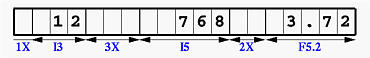# Horizontal Spacing: nXTo skip the next n positions, use the X edit descriptor. The general form of this descriptor is as follows:

nX

• The next n positions are skipped. The X edit descriptor can be used for both input and output. For output, the next n positions are skipped and the content there is unchanged. For input, it simply skips the n positions.
• Unlike edit descriptors I, F, E, L and A, the number of positions is placed before the edit descriptor. Do not write Xn.
• Edit descriptor X cannot be used with repetition indicator directly and n is not a repetition indicator.
• The X edit descriptor is only for skipping positions and does not read and write any values.### Examples

• Consider the following input:
```         1    1    2
....5....0....5....0
123   456   789  012
```
```INTEGER          :: a
REAL             :: b
CHARACTER(LEN=4) :: c

```
The first edit descriptor is 2X, meaning that the first two positions (i.e., 1 and 2) should be skipped. Then, I4 is for variable a. The next four positions, which contain 3 and three spaces, are for a, and as a result, a receives 3 if spaces are ignored or 3000 if spaces are treated as zeros. The next edit descriptor is 3X, meaning that the next three positions (i.e., 4, 5 and 6) should be skipped. This is followed by F5.2, which is used to read a REAL number for variable b. Since these five positions contain three spaces, 7 and 8, variable c receives 0.78. The next edit descriptor is 2X, which causes the next two positions (i.e., 9 and space) skipped. Finally, A is used to read a CHARACTER string for variable c. Since c has length 4, this A is equivalent to A4. Therefore, c receives the next four characters " 012".
• Consider the following WRITE statement:
```CHARACTER(LEN=30) :: FMT = "(1X,I3,3X,I5,2X,F5.2)"
INTEGER           :: a = 12
INTEGER           :: b = 768
REAL              :: c = 3.715